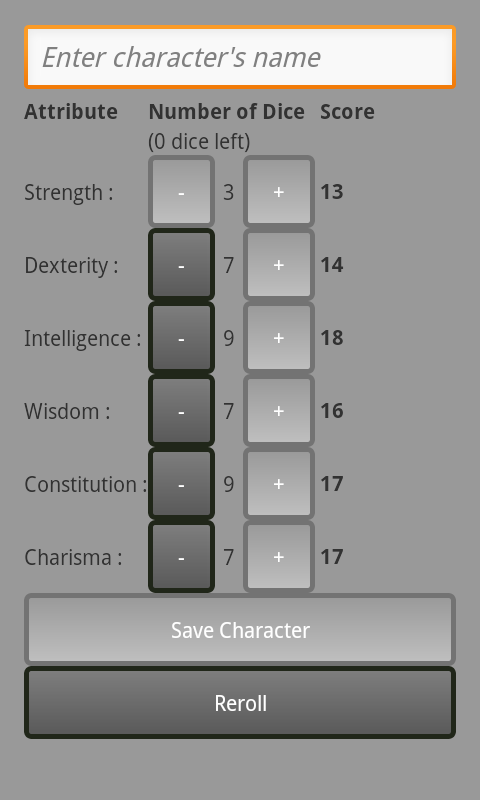# Formula for computing ability modifiersHere’s something that should interest… almost no one… but I’m sharing nonetheless. Since the game is based on ability scores, I need to compute an ability modifier for a given score. One could brute force it with a table, but I think the following is more elegant:

```private static int abilityModifer(int abilityScore) {
return (abilityScore / 2 - 5);
}```

Given an integer ability score, this Java method returns an integer ability modifier. Easy.

It might be helpful to note that while (x / 2) – 5 is equivalent to (x – 10) / 2, if you go with the latter, which is how I started, the code gets a little more complicated:

```private static int abilityModifer(int abilityScore) {
return (int) Math.floor((float) (abilityScore - 10) / 2);
}```

The problem is with the rounding. The trick here, that took me no small amount of time to figure out, is that you need to cast the `(abilityScore - 10) / 2` argument to a float before passing it through `Math.floor()`. If not, the negative modifiers aren’t calculated correctly because the argument gets implicitly cast to an int which, for ability scores less than 10, rounds towards zero, which is the wrong direction. `Math.floor()` will round the float toward negative infinity, which is what you want.## Author: Ĕlāhā

Lord and Creator of the Mortal Wayfare universe